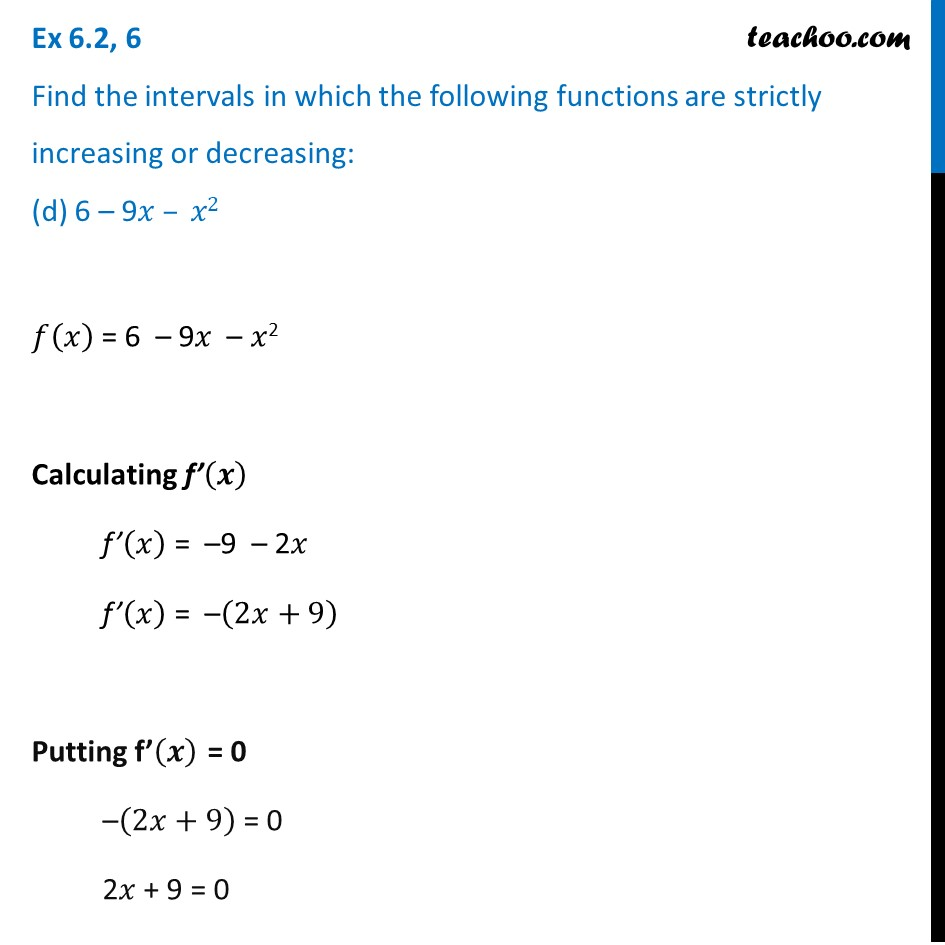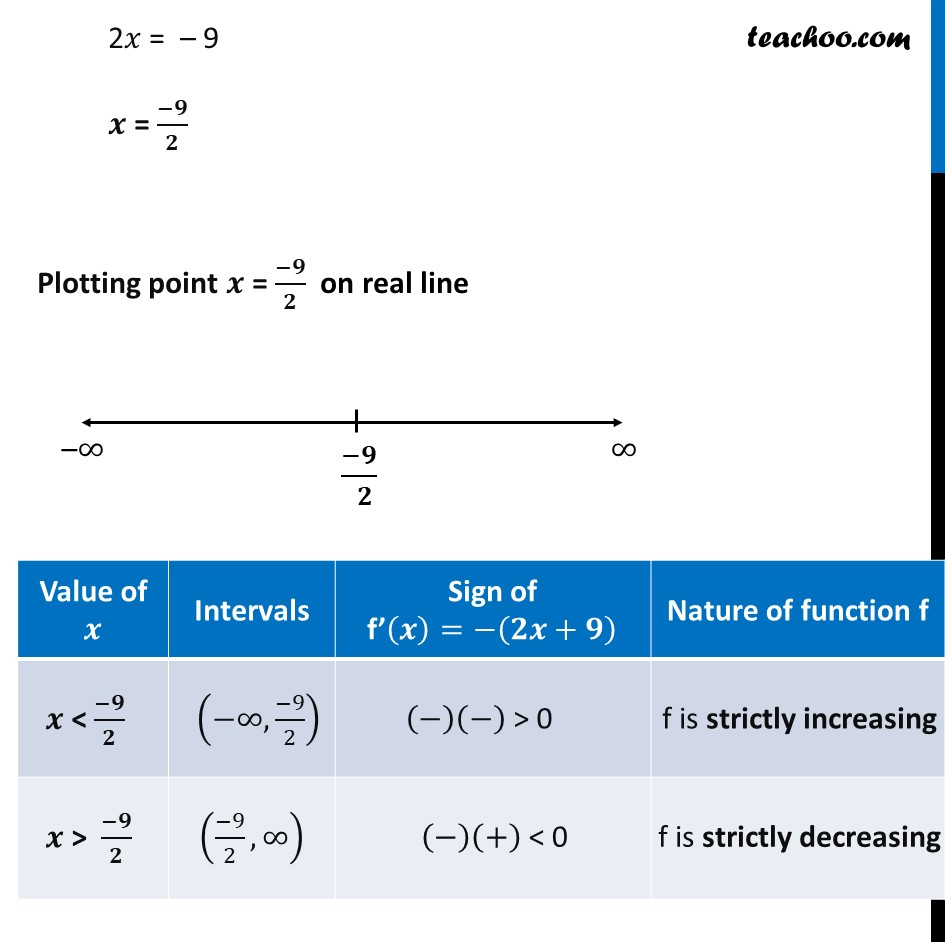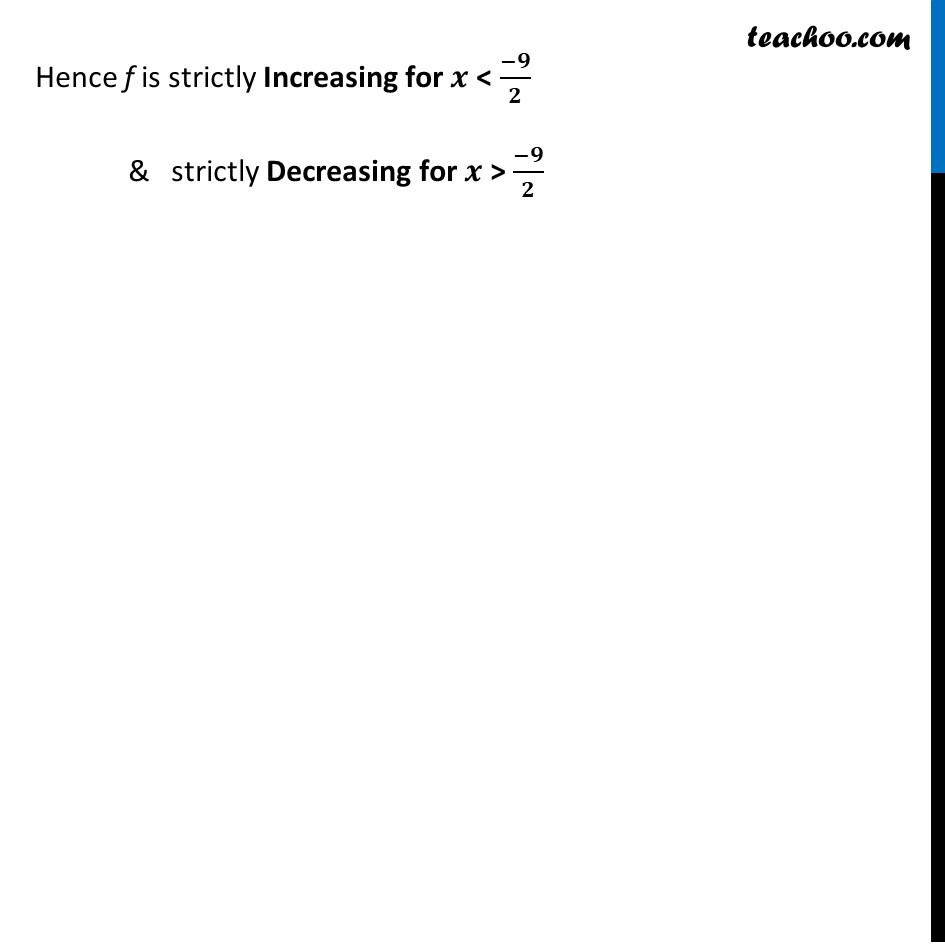Ex 6.2

Chapter 6 Class 12 Application of Derivatives
Serial order wiseLearn in your speed, with individual attention - Teachoo Maths 1-on-1 Class

### Transcript

Ex 6.2, 6 Find the intervals in which the following functions are strictly increasing or decreasing: (d) 6 – 9𝑥 – 𝑥2 𝑓(𝑥) = 6 – 9𝑥 – 𝑥2 Calculating f’(𝒙) f’(𝑥) = –9 – 2𝑥 f’(𝑥) = –(2𝑥+9) Putting f’(𝒙) = 0 –(2𝑥+9) = 0 2𝑥 + 9 = 0 2𝑥 = – 9 𝒙 = (−𝟗)/𝟐 Plotting point 𝒙 = (−𝟗)/𝟐 on real line Hence f is strictly Increasing for 𝒙 < (−𝟗)/𝟐 & strictly Decreasing for 𝒙 > (−𝟗)/𝟐